# Roman Numerals

## NUMBERS AND OPERATIONSRoman numerals is a numbering system that developed in Ancient Rome and was used throughout the Roman Empire maintained after his disappearance.

The Roman numeration system uses uppercase letters (from the Latin alphabet) as symbols to represent certain values, leading to the interpretation of numbers as the result of letter combinations following an additive and subtractive system, that is, using both addition and subtraction.

WHAT DO WE USE ROMAN NUMBERS FOR?

Today we still use Roman numerals on countless occasions and, below, we will show you some of the examples:

We use Roman numerals to name kings and queens (Felipe VI of Spain, Isabel II of the United Kingdom), the popes (Francisco I), to identify centuries (21st century), anniversaries, congresses, competitions, awards, the Olympic games, the chapters of a book, the acts and scenes of the plays... and we even use them on the clocks.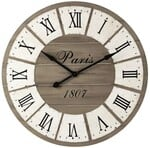ANCIENT ROMAN EMPIRE

Could you explain what a Roman circus is? And an aqueduct? And what are the Roman baths? All these words come from the ancient Roman Empire.

Did you know that the Romans created causeways all over the Mediterranean? In Spain, the most important carriageway was the Via Augusta and it measured more than 1,500 kilometers connecting the Pyrenees with Cádiz.

Select the video to learn more about the Roman Empire: the founding of Rome, the Republic, the Roman emperors, the estates which was divided into Roman society, the amphitheaters, the legion...

SPANISHENGLISHEQUIVALENCES BETWEEN ROMAN AND ARABIC NUMBERS

Do you know what Roman numerals are and what are they for? Can you tell how much each letter is worth? Do you know all his writing rules? Don't worry, we provide you with templates in PDF format so that you can download and print all Roman numerals from 0 to 2,000 as well as the equivalence between the Indo-Arabic and Roman numerals.

#### Recognize the value of letters in the Roman Numeration System: I - V - X - L - C - D - M

The Roman Numeral System is made up of 7 capital letters where each one of them has its own numerical value.

The natural numbers are built by joining these symbols, where, based on a set of rules, each of the letters will add or subtract.

Very important! Remember that Roman numerals are read and written from left to right as well as from highest to lowest value.

SPANISHENGLISHVALUE OF ROMAN LETTERSCuriosity: Roman numerals are written with letters of the Roman alphabet (also known as "Latin alphabet"), but they originally came from the Etruscans (people of Antiquity originating in the province of Tuscany, in northern Italy), who used the symbols I, Λ, X, Ψ, 8 and ⊕ to represent I, V, X, L, C and M.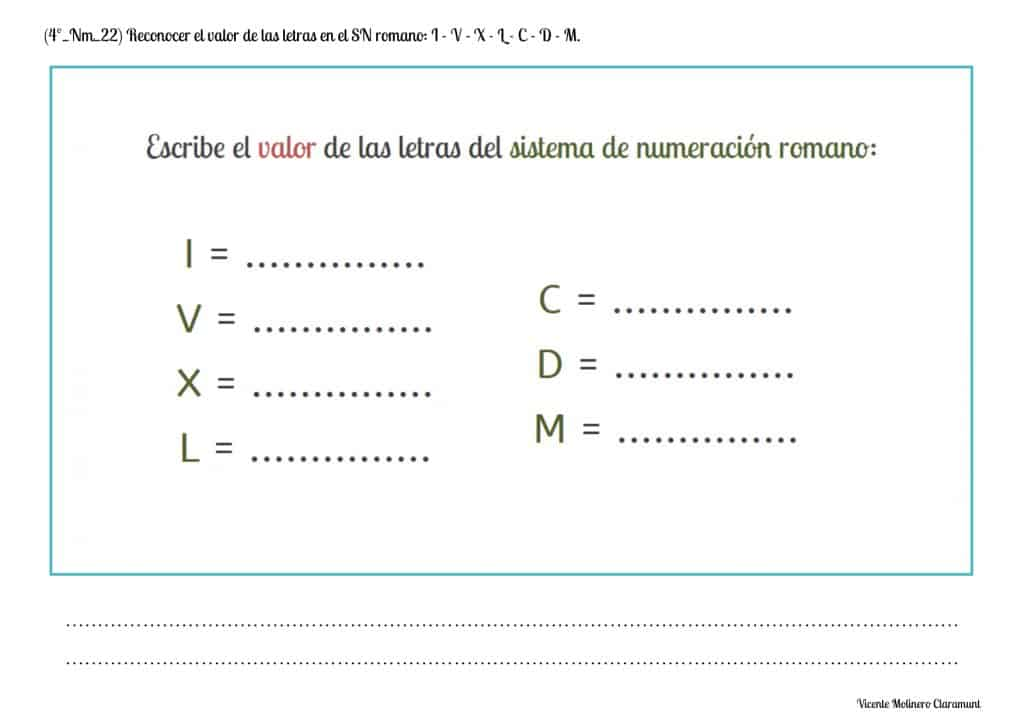#### Know and apply the rules of the Roman Numeral System: Addition rule

We propose some activities in PDF format together with the explanation of the addition rule:

"A letter written to the right of another of equal or greater value, adds its value to that letter"

Very important! The symbols V (value 5), L (value 50) and D (value 500) always add up, that is, they can never be to the left of a higher value symbol to subtract .

SPANISHENGLISHLet's see the explanation using the example of the number 95:

If we represent the number 95 using the letters VC it would be incorrect (since the letter V cannot be to the left of a higher value number such as, in this case, the letter C which equals 100). Therefore, we will represent the number 95 using the XCV symbols (100 - 10 + 5)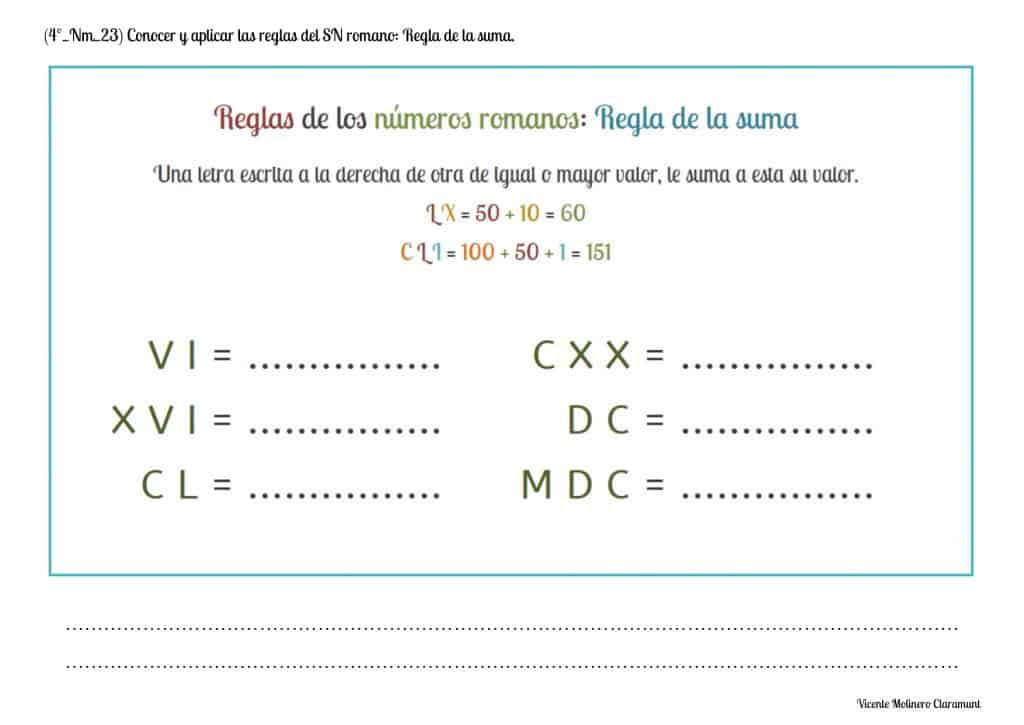#### Know and apply the rules of the Roman Numeral System: Subtraction rule

"The letters I, X and C written to the left of each of the two letters with the highest value that follow, subtract its value from that letter"

Rules for correctly writing Roman numerals following the subtraction rule:

- The symbol I can only subtract V (value 5) and X (value 10)

- The symbol X can only subtract L (value 50) and C (value 100)

- The symbol C can only subtract D (value 500) and M (value 1,000)

SPANISHENGLISHSUBTRACTION RULE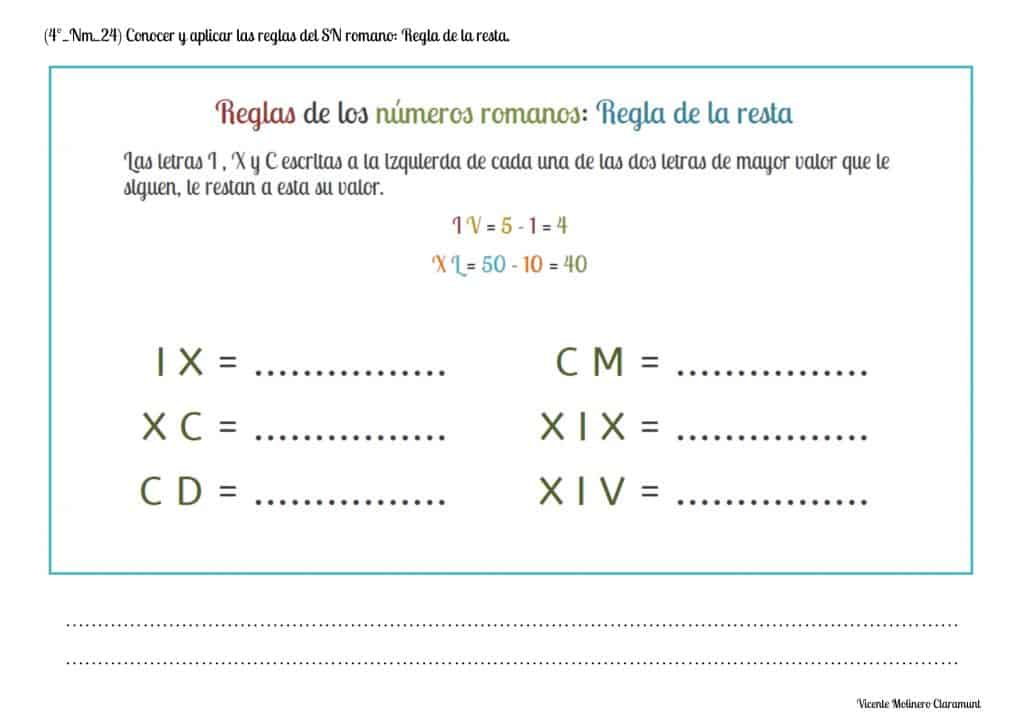#### Know and apply the rules of the Roman Numeral System: Repetition rule

We propose some activities ready to print together with the explanation of the repetition rule:

"The letters I, X, C and M can be repeated two and three times and always add up; on the other hand, the letters V, L and D cannot be repeated"

Very important: The V, the L and the D cannot be duplicated because other letters ("X", "C" and "M") represent their duplicate value:

The number 10 is represented by the letter X since writing it "VV" (5 + 5) would be incorrect. Nor can we write "LL" (50 + 50) to represent 100 (we use the letter C) or repeat "DD" (500 + 500) to represent 1,000 since we use the symbol M

SPANISHENGLISHREPETITION RULE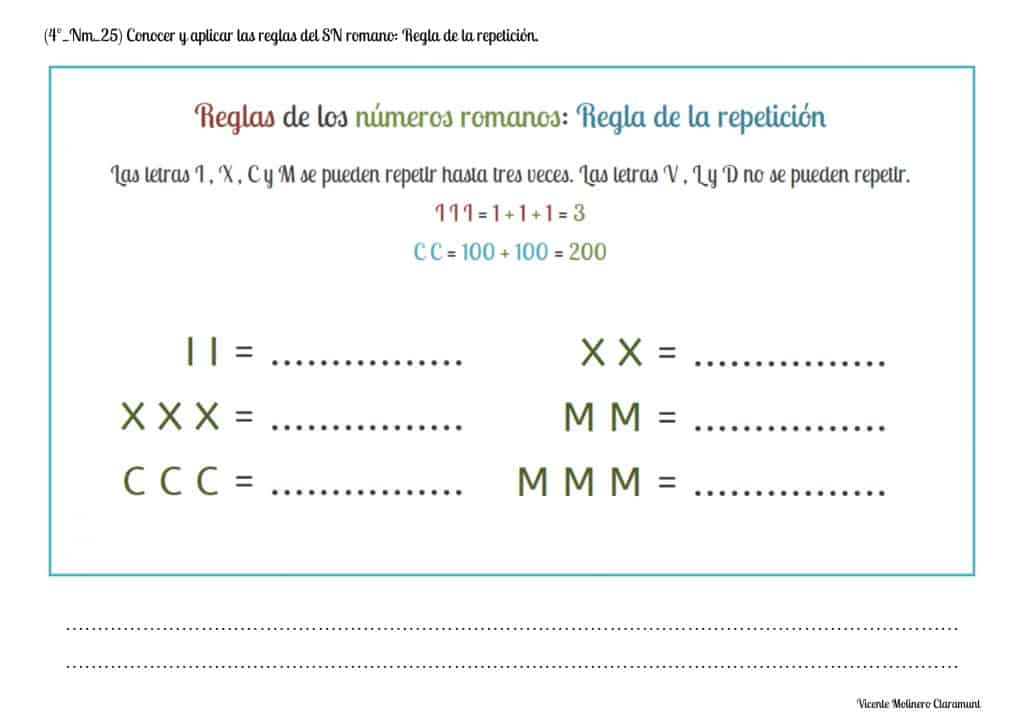#### Know and apply the rules of the Roman Numeral System: Multiplication rule

We provide you with exercises accompanied by the explanation of the multiplication rule that is used to write numbers equal to or greater than 4,000:

"An upper bar above a letter or group of letters multiplies its value by a thousand"

SPANISHMULTIPLICATION RULE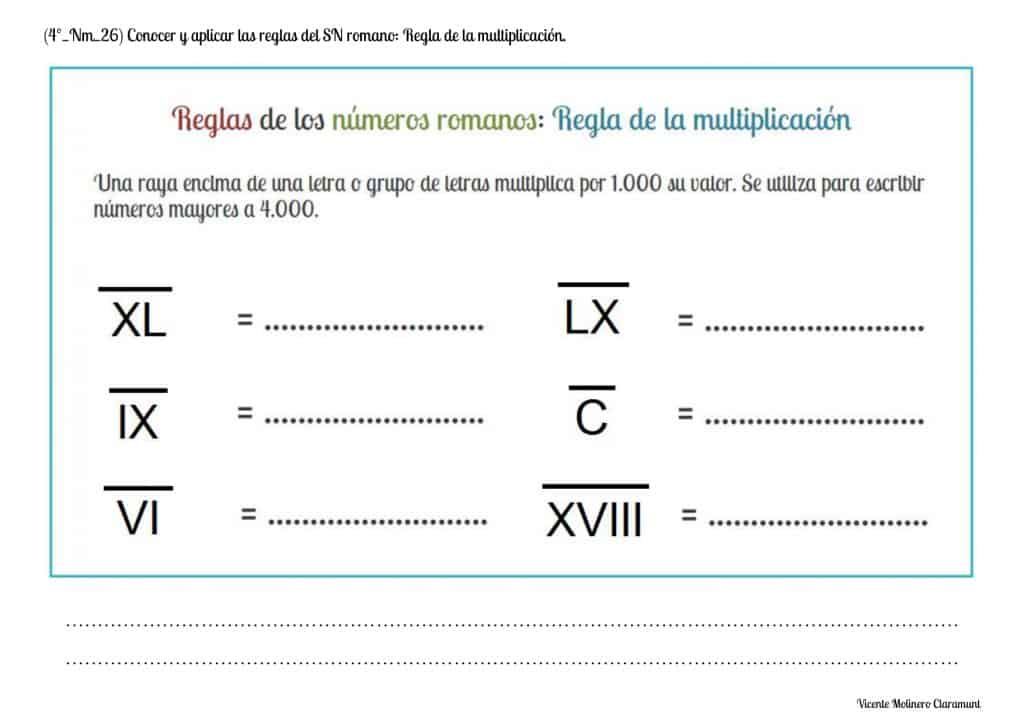#### Read and write numbers in the Roman Numeral System

ROMAN NUMBERS FROM 1 TO 10

We explain how to read and write the Roman numerals from 1 to 10 and we accompany you with our fantastic activities in PDF format as well as a series of educational games.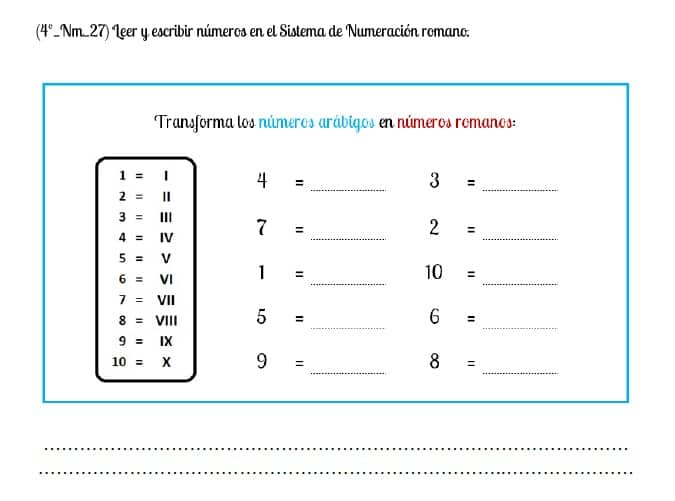ROMAN NUMBERS FROM 1 TO 20

We teach you how to correctly read and write the different Roman numerals from 1 to 20 and we propose a variety of downloadable exercises along with interactive games to that you practice your learning.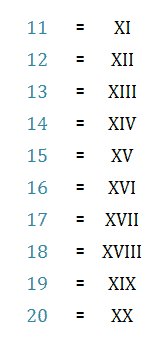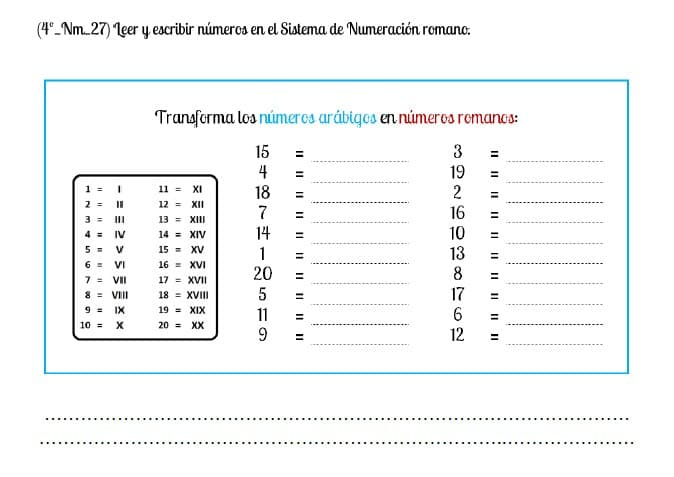ROMAN NUMBERS FROM 1 TO 30

Practice combining the letters of the Roman numerals and learn to write them easily from 1 to 30. Also enjoy our printable educational worksheets as well as the online activities that we have expressly selected for you.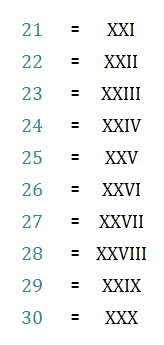ROMAN NUMBERS FROM 1 TO 50

Keep trying and you will learn to read and write Roman numerals from 1 to 50. Don't forget to solve our PDF worksheets and the educational games that we have prepared to boost your learning.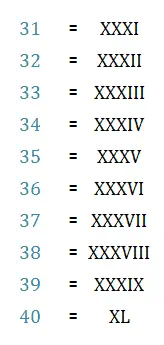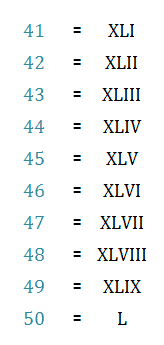ROMAN NUMBERS FROM 1 TO 100

We wish to share with you an ordered table with all numbers from 1 to 100 written both in their Indo-Arabic form and using the Roman numeral system.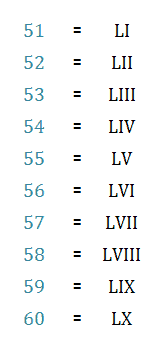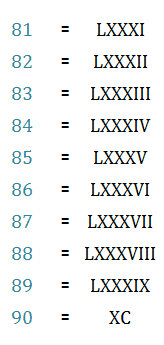ROMAN NUMBERS FROM 100 TO 500

We show you how to write Roman numerals from 100 to 500 and we accompany it with some tasks in PDF format and an entertaining educational activity.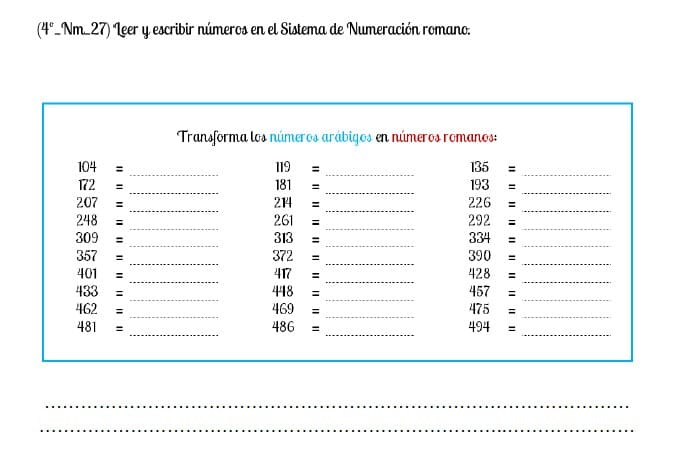ROMAN NUMBERS FROM 1 TO 1,000

The time has come to prove that you deserve to become the hero of a Roman epic... achieve glory by correctly typing any natural number from 1 to 1,000. Are you ready for battle?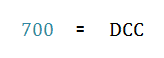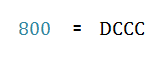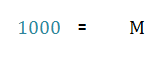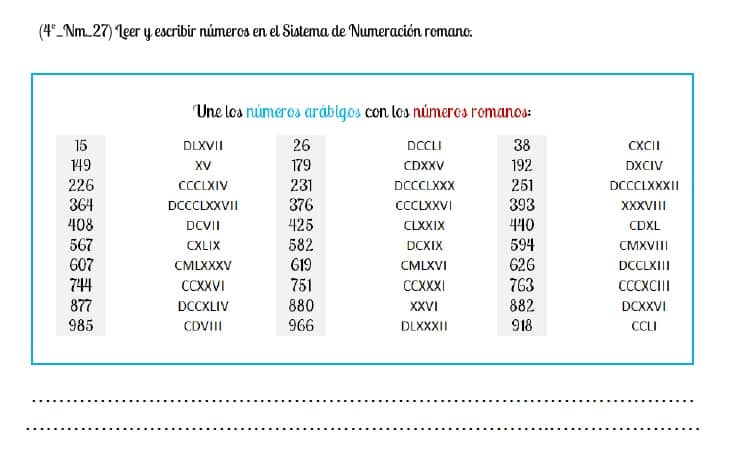ROMAN NUMBERS UP TO THOUSANDS

Do you know how to write the Roman numerals from 1 to 10,000? And from 1 to 100,000? We teach you the basic rules that you must know to successfully face the reading and writing of any Roman numeral up to 999,999. Come on centurion!

Rule for writing Roman numerals up to 3,999

We will use the rule of repetition using the letter "M" to represent 1,000 (M), 2,000 (MM) and 3,000 (MMM).

Example: MMDCCCLXXIX = 2,000 (MM) + 800 (DCCC) + 70 (LXX) + 9 (IV) = 2,879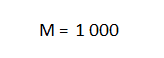Rule for writing Roman numerals from 4,000

We cannot repeat, in a row, more than three times a letter of the Roman numeration system (rule of repetition) so the number 4,000 cannot be represented with 4 letters "M". The solution is to draw a top bar above a letter or group of letters so that its value is multiplied by a thousand.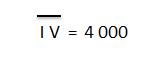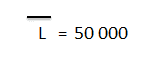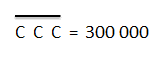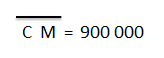ROMAN NUMBERS UP TO MILLIONS

And now the most difficult one... could you write any Roman numeral using the millions? Pay attention to the writing rules and shine like a gladiator in the Colosseum.

Rule for writing Roman numerals up to 3,999,999

Again we will use the symbol "M" and apply the rule of repetition. But now, as a differentiating element, we will draw a top bar so that each of the letters (M, MM and MMM) are multiplied by 1,000

For example, the number 2,000,000 arises from multiplying MM (value 2,000) by 1,000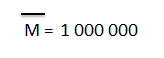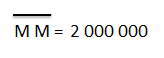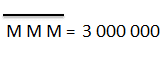Rule for writing Roman numerals from 4,000,000

To represent Roman numerals equal to or greater than 4,000,000, we will draw an upper horizontal double line over the group of letters that we wish to multiply by 1,000,000

Remember that each of the horizontal lines that we write on the letters multiplies the value of that letter by 1,000. In this case, as it is a double upper bar, it will multiply the value of the letters 2 times by 1,000: 1,000 x 1,000 = 1,000,000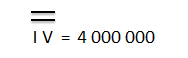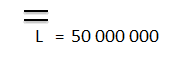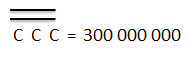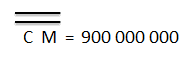ROMAN NUMBERS UP TO BILLIONS

This time we are going to teach you to write very large amounts using Roman numerals, specifically, we will show you how to write the billions from the Roman numeral system.

Rule for writing Roman numerals up to 3,999,999,999

If we want to write a billion, two billion, or three billion; we simply have to draw a double upper bar over each of the letters (M, MM and MMM) so that they are multiplied by 1,000,000

For example, the number 3,000,000,000 comes from multiplying MMM (value 3,000) by 1,000,000 (double horizontal bar, that is, 1,000 x 1,000).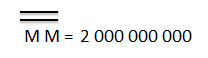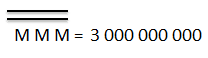Rule for writing Roman numerals from 4,000,000,000

To represent Roman numerals equal to or greater than 4,000,000,000 we will draw a triple upper horizontal line over the group of letters that we wish to multiply by 1,000,000,000

Remember that each of the horizontal lines that we write on the letters multiplies the value of that letter by 1,000. In this case, being a upper triple bar, it will multiply the value of the letters 3 times by 1,000: 1,000 x 1,000 x 1,000 = 1,000,000,000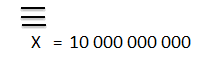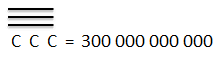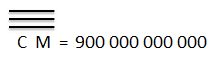#### Classify numbers from the Roman Numeral System: Greater than - Less than - Equal

After learning how to write any Roman numeral correctly, we present you a set of activities so that you can also compare any two Roman numerals indicating which is the greater, the lower or if they are equivalent.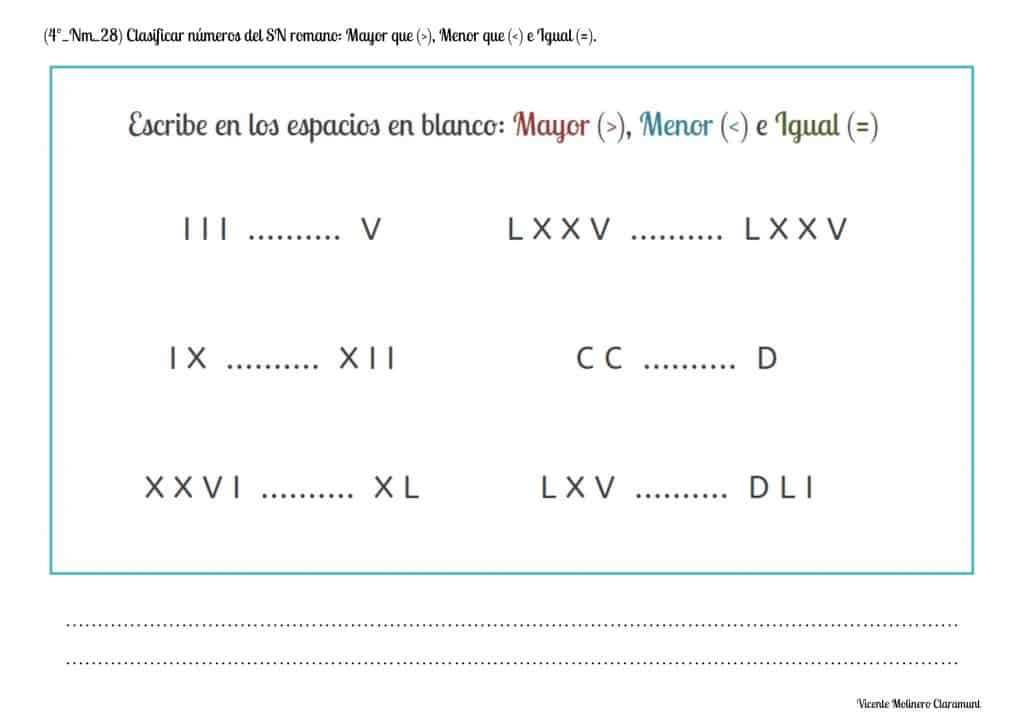#### Identify the largest and smallest number in a series of numbers from the Roman Numeral System

We teach you to identify which is the greatest number and the smallest of a series of random Roman numerals from the downloadable exercises that we have prepared.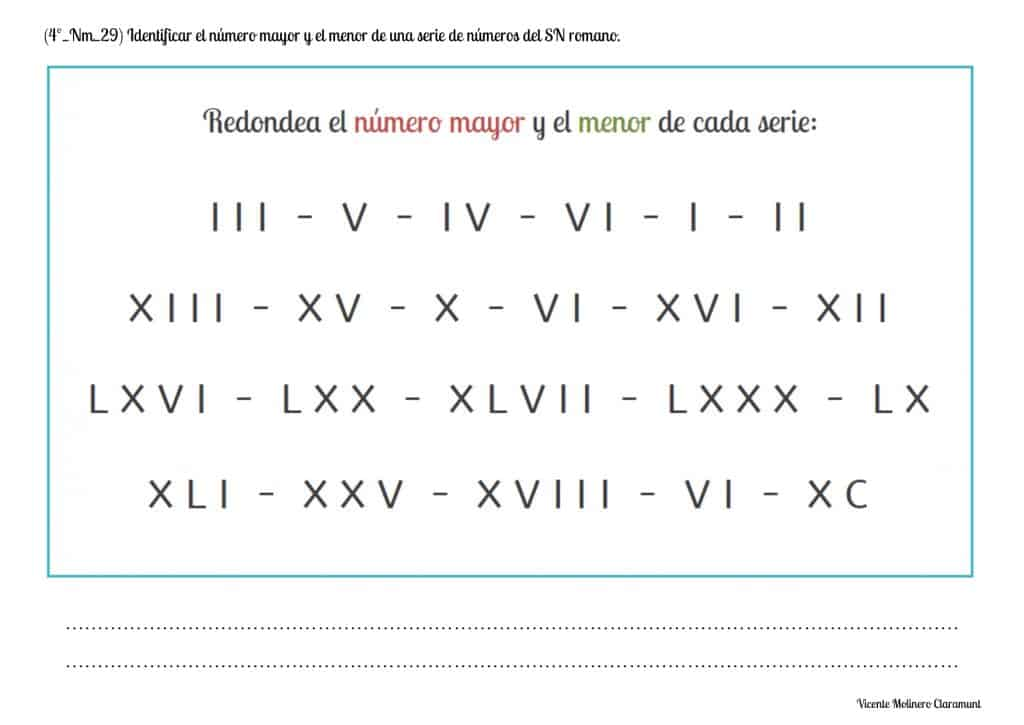#### Classify numbers of the Roman Numeration System: Before - After numbers

We provide you with worksheets in PDF where you have to join the Roman numerals of the central column with their previous number (left) and their next (right).#### Write the numbers of the Roman Numeration System before and after a given one

We instruct you in an learning where we present you some Roman numerals that you must identify and then write their previous number (left) and their after number (right).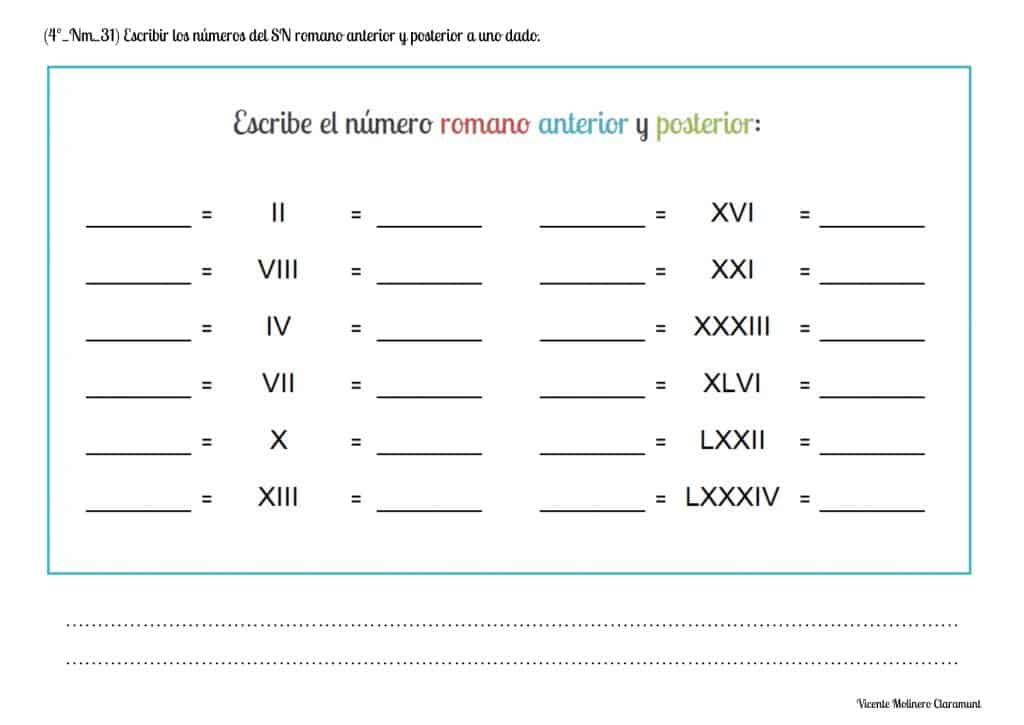Select more exercises, worksheets and activities of Mathematics for each of the 4 learning blocks of the Educational Curriculum ("Numbers and Operations", "Quantities and Measurements", "Geometric and Spatial Reasoning" and "Data Analysis and Probability") aimed at improving the logical-mathematical competencies and skills that are developed throughout "Grade 4" or "Year 5":